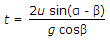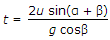# Mechanical Engineering - Engineering Mechanics

### Exercise :: Engineering Mechanics - Section 1

11.

A smooth cylinder lying on its convex surface remains in __________ equilibrium.

 A. stable B. unstable C. neutral

Explanation:

No answer description available for this question. Let us discuss.

12.

Coefficient of friction is the ratio of the limiting friction to the normal reaction between the two bodies.

 A. Yes B. No

Explanation:

No answer description available for this question. Let us discuss.

13.

Moment of inertia of a circular section about an axis perpendicular to the section is

 A. πd3/16 B. πd3/32 C. πd4/32 D. πd4/64

Explanation:

No answer description available for this question. Let us discuss.

14.

The time of flight (t) of a projectile on an upward inclined plane is(where u = Velocity of projection, α = Angle of projection, and β = Inclination of the plane with the horizontal.)

 A.B.C.D.Explanation:

No answer description available for this question. Let us discuss.

15.

The unit of angular acceleration is

 A. N-m B. m/s C. m/s2 D. rad/s2